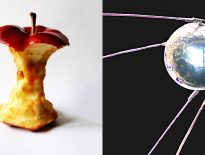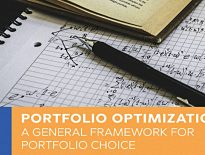# Sharpe RatioToday’s post is about the Sharpe Ratio, which compares the returns from an investment to their volatility / variability.

###### Sharpe Ratio

The Sharpe Ratio (SR) is a measure of return per unit risk (or rather, volatility), named after the Stanford professor (and Nobel laureate) William F. Sharpe who invented it in 1966.

• It measures the compensation (return) than you get for taking on an additional unit of risk (volatility) above the risk-free asset.

The formula is:

SR = ( Rp – Rf ) / σp

Here, Rp is the return from a portfolio and Rf is the risk-free rate (usually on short-dated government bonds / bills).

• Rp – Rf is known as the risk premium.
• σp (sigma p) is the standard deviation of the portfolio, a measure of the volatility of returns.

If two portfolios have the same expected (or historical) returns, then the one with the lower SR is the better choice.

• The same applies in reverse – within two portfolios with the same SR, the one with the higher returns is (obviously) the better.

Note that this is not the same as saying that investors should maximise their SR.

• The primary purpose of investment is to achieve a required return.

For example, I need to target my SWR plus inflation, which over the long-run is approximately 3.25% + 2.5% = 5.75% pa.

• Portfolios returning less than 5.75% pa (gross / nominal) will not meet my needs.

Investors should instead maximise SR for a given target Rp.

###### Common values

A positive SR means that returns are above the risk free rate, whereas a negative SR means that returns are below the risk-free rate.

An SR value between 0 and 1 means that the excess returns come with a lot of excess risk (volatility), compared to a short-term bond.

An SR above 1 means that the  excess returns come with less excess risk than expected.

The Sharpe ratios of individual asset classes are usually around 0.2 to 0.3 (over the long-run).

###### Predictive use

Some studies have found predictive value in historic SRs (of hedge funds, for example).

• The implication is that investors should avoid funds with low Sharpe Ratios as these are likely to underperform in the future.

Note that the persistency of Sharpe Ratios is likely to be affected by significant market turmoil (crashes).

Note also that SR persistency is not the same as consistent high returns.

• Returns might fall but the SR could be preserved by a corresponding fall in volatility.
###### Risk

The first SR issue we must deal with is that of risk.

• The Sharpe Ratio was originally known as the reward-to-variability ratio, which I think is a more accurate name (though it’s a bit of a mouthful).

As we have discussed many times, Volatility is not Risk.

Equities provide very volatile returns, but they are not particularly risky over the long-term.

• More importantly, high-return, low-volatility assets are not available.

So to achieve the returns we need, we must accept some volatility.

• Not taking on that volatility is the real risk.

This does not invalidate the usefulness of the Sharpe Ratio, but the SR is easier to understand if you think about it in terms of volatility.

It should be noted that the SR treats all volatility as equal, whereas investors are mostly concerned about downside volatility (losses).

###### Returns

Returns and risk (volatility) are broadly correlated, though the free lunch of diversification using assets with less than perfect correlations can compensate for this.

• Well-diversified portfolios will have higher SRs.

It’s important to check whether apparently high returns are being generated through the use of:

1. high-volatility assets (eg. AIM shares)
2. concentrated portfolios
3. leverage

All of these can improve returns, at the risk of impacting the Sharpe Ratio.

• Leverage doesn’t affect the SR directly (since the numerator and denominator are inflated to the same degree).
• But the consequences of a large drawdown in a leveraged portfolio (margin calls, forced sales etc) will ultimately impact the investor’s SR.
###### Illiquidity

Looking at the opposite end of the spectrum, illiquid assets (which are not priced / traded every day) will appear to have smoother returns than they really do.

• This in turn will lead to misleadingly good Sharpe Ratios. (( For the same reason, Ponzi schemes – where the consistent returns are imaginary – will typically have high Sharpe Ratios ))

Smoothed-return assets (like with-profits schemes) will also have misleadingly high SRs.

Note that in some senses this is a philosophical point – if you can’t get out of an illiquid asset, then your personal experience of the returns will in fact show low volatility.

• Cashflows don’t lie, and the use of illiquid assets can be see as the equivalent of Ulysses lashing himself to the mast to avoid the temptations of the Sirens.
###### Abnormal distributions

The use of standard deviation in the SR formula implies that the returns from the portfolio follow a normal (Gaussian) distribution.

This is almost never the case, and for some investments the distribution of returns can be very far from normal.

• Such investments can produce misleading SRs.

Things that the SR ignores by assuming a normal distribution include:

• asymmetry of the distribution of returns (skew)
• fat tails / extreme returns (kurtosis)
• non-linear risks (typically from derivatives like options and warrants)
###### Variations

In recent years the risk free rate has been close to zero and so many commentators have ignored Rf in the calculation.

Another common variation is to substitute a benchmark portfolio as a reference point instead of the risk-free rate.

• The ratio is then known as the information ratio.

It’s important to choose an appropriate benchmark, such that the beta of the portfolio relative to the benchmark is close to one.

• The same applies to the use of a peer group as a benchmark – the choice of appropriate peers is crucial.
###### Alternatives

Alternatives to the Sharpe ratio include:

1. The Treynor ratio
• This compares the return on a portfolio to its systematic risk by dividing the excess return by the beta of the portfolio.
• This makes it most useful for well-diversified portfolios (in contrast to the information ratio).
2. The Sortino ratio
• This uses a minimum acceptable return (MAR) instead of the risk-free rate.
• And only looks at the standard deviation of returns below that MAR (ie. upside volatility is ignored).
3. VaR (Value at Risk)
• This is a different approach to evaluating portfolios, which looks at the maximum loss for a given level of confidence.
• Eg. the portfolio has a 95% chance of not losing more that 10% in a year.
4. Other less-commonly used methods for evaluating portfolios include:
• non-portfolio benchmarks (especially inflation, but also long duration risk-free assets like index-linked bonds),
• the Omega ratio (the probability weighted ratio of gains versus losses for some threshold return target).
###### Conclusions

The Sharpe Ratio is easy to calculate, and is a useful way to compare portfolios with similar returns, or to illustrate the trade-off between returns and volatility.

Just remember not to maximise for the SR, but instead find the portfolio that produces your target return with the lowest volatility.

• Which I guess means that I think that the Sortino ratio is better.

The Omega function looks good, too, but it is little-used and not easy to calculate.

Until next time.

### Mike RawsonMike is the owner of 7 Circles, and a private investor living in London. He has been managing his own money for 39 years, with some success.

## Sharpe Ratio

by Mike Rawson time to read: 4 min